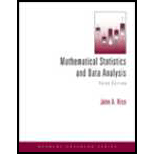# Compute the probability of k suicides in a year for k = 0 , 1 , 2 , … . Find the most probable number of suicides.### Mathematical Statistics and Data A...

3rd Edition
John A. Rice
Publisher: Cengage Learning
ISBN: 9781111793715### Mathematical Statistics and Data A...

3rd Edition
John A. Rice
Publisher: Cengage Learning
ISBN: 9781111793715

#### Solutions

Chapter 2.5, Problem 30P

a.

To determine

## Compute the probability of k suicides in a year for k=0,1,2,….Find the most probable number of suicides.

b.

To determine

### Want to see the full answer?

Check out a sample textbook solution.

### Want to see this answer and more?

Experts are waiting 24/7 to provide step-by-step solutions in as fast as 30 minutes!*

*Response times may vary by subject and question complexity. Median response time is 34 minutes for paid subscribers and may be longer for promotional offers.